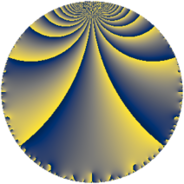# Properties

 Label 147.4.eLevel $147$ Weight $4$ Character orbit 147.e Rep. character $\chi_{147}(67,\cdot)$ Character field $\Q(\zeta_{3})$ Dimension $40$ Newform subspaces $14$ Sturm bound $74$ Trace bound $5$

# Related objects

## Defining parameters

 Level: $$N$$ $$=$$ $$147 = 3 \cdot 7^{2}$$ Weight: $$k$$ $$=$$ $$4$$ Character orbit: $$[\chi]$$ $$=$$ 147.e (of order $$3$$ and degree $$2$$) Character conductor: $$\operatorname{cond}(\chi)$$ $$=$$ $$7$$ Character field: $$\Q(\zeta_{3})$$ Newform subspaces: $$14$$ Sturm bound: $$74$$ Trace bound: $$5$$ Distinguishing $$T_p$$: $$2$$, $$5$$

## Dimensions

The following table gives the dimensions of various subspaces of $$M_{4}(147, [\chi])$$.

Total New Old
Modular forms 128 40 88
Cusp forms 96 40 56
Eisenstein series 32 0 32

## Trace form

 $$40 q - 4 q^{2} - 6 q^{3} - 60 q^{4} + 8 q^{5} + 24 q^{6} - 156 q^{8} - 180 q^{9} + O(q^{10})$$ $$40 q - 4 q^{2} - 6 q^{3} - 60 q^{4} + 8 q^{5} + 24 q^{6} - 156 q^{8} - 180 q^{9} - 46 q^{10} + 40 q^{11} - 72 q^{12} + 4 q^{13} + 84 q^{15} - 164 q^{16} + 132 q^{17} - 36 q^{18} - 218 q^{19} - 872 q^{20} - 180 q^{22} + 208 q^{23} - 54 q^{24} - 686 q^{25} + 466 q^{26} + 108 q^{27} + 920 q^{29} + 216 q^{30} - 348 q^{31} + 1050 q^{32} - 150 q^{33} + 552 q^{34} + 1080 q^{36} + 550 q^{37} - 350 q^{38} + 42 q^{39} - 42 q^{40} - 1208 q^{41} - 2076 q^{43} - 1112 q^{44} + 72 q^{45} - 1076 q^{46} - 240 q^{47} + 1872 q^{48} - 920 q^{50} + 48 q^{51} + 260 q^{52} + 2884 q^{53} - 108 q^{54} + 1972 q^{55} - 1140 q^{57} + 418 q^{58} + 1128 q^{59} - 1938 q^{60} - 188 q^{61} - 3636 q^{62} + 2664 q^{64} - 336 q^{65} + 156 q^{66} - 2290 q^{67} + 2028 q^{68} + 1800 q^{69} + 376 q^{71} + 702 q^{72} + 1350 q^{73} + 1534 q^{74} - 738 q^{75} + 4712 q^{76} - 108 q^{78} + 248 q^{79} + 388 q^{80} - 1620 q^{81} - 956 q^{82} - 1992 q^{83} + 2264 q^{85} + 4670 q^{86} - 1050 q^{87} + 6198 q^{88} + 2672 q^{89} + 828 q^{90} - 10360 q^{92} - 3006 q^{93} - 3204 q^{94} - 928 q^{95} - 1914 q^{96} - 1044 q^{97} - 720 q^{99} + O(q^{100})$$

## Decomposition of $$S_{4}^{\mathrm{new}}(147, [\chi])$$ into newform subspaces

Label Dim $A$ Field CM Traces $q$-expansion
$a_{2}$ $a_{3}$ $a_{5}$ $a_{7}$
147.4.e.a $2$ $8.673$ $$\Q(\sqrt{-3})$$ None $$-4$$ $$-3$$ $$-18$$ $$0$$ $$q-4\zeta_{6}q^{2}+(-3+3\zeta_{6})q^{3}+(-8+8\zeta_{6})q^{4}+\cdots$$
147.4.e.b $2$ $8.673$ $$\Q(\sqrt{-3})$$ None $$-4$$ $$-3$$ $$-4$$ $$0$$ $$q-4\zeta_{6}q^{2}+(-3+3\zeta_{6})q^{3}+(-8+8\zeta_{6})q^{4}+\cdots$$
147.4.e.c $2$ $8.673$ $$\Q(\sqrt{-3})$$ None $$-4$$ $$3$$ $$4$$ $$0$$ $$q-4\zeta_{6}q^{2}+(3-3\zeta_{6})q^{3}+(-8+8\zeta_{6})q^{4}+\cdots$$
147.4.e.d $2$ $8.673$ $$\Q(\sqrt{-3})$$ None $$-4$$ $$3$$ $$18$$ $$0$$ $$q-4\zeta_{6}q^{2}+(3-3\zeta_{6})q^{3}+(-8+8\zeta_{6})q^{4}+\cdots$$
147.4.e.e $2$ $8.673$ $$\Q(\sqrt{-3})$$ None $$1$$ $$-3$$ $$12$$ $$0$$ $$q+\zeta_{6}q^{2}+(-3+3\zeta_{6})q^{3}+(7-7\zeta_{6})q^{4}+\cdots$$
147.4.e.f $2$ $8.673$ $$\Q(\sqrt{-3})$$ None $$1$$ $$3$$ $$-12$$ $$0$$ $$q+\zeta_{6}q^{2}+(3-3\zeta_{6})q^{3}+(7-7\zeta_{6})q^{4}+\cdots$$
147.4.e.g $2$ $8.673$ $$\Q(\sqrt{-3})$$ None $$3$$ $$-3$$ $$-18$$ $$0$$ $$q+3\zeta_{6}q^{2}+(-3+3\zeta_{6})q^{3}+(-1+\zeta_{6})q^{4}+\cdots$$
147.4.e.h $2$ $8.673$ $$\Q(\sqrt{-3})$$ None $$3$$ $$3$$ $$-3$$ $$0$$ $$q+3\zeta_{6}q^{2}+(3-3\zeta_{6})q^{3}+(-1+\zeta_{6})q^{4}+\cdots$$
147.4.e.i $2$ $8.673$ $$\Q(\sqrt{-3})$$ None $$3$$ $$3$$ $$18$$ $$0$$ $$q+3\zeta_{6}q^{2}+(3-3\zeta_{6})q^{3}+(-1+\zeta_{6})q^{4}+\cdots$$
147.4.e.j $4$ $8.673$ $$\Q(\sqrt{2}, \sqrt{-3})$$ None $$-2$$ $$-6$$ $$20$$ $$0$$ $$q+(-1+\beta _{1}-\beta _{2})q^{2}+3\beta _{2}q^{3}+(-2\beta _{1}+\cdots)q^{4}+\cdots$$
147.4.e.k $4$ $8.673$ $$\Q(\sqrt{2}, \sqrt{-3})$$ None $$-2$$ $$6$$ $$-20$$ $$0$$ $$q+(-1+\beta _{1}-\beta _{2})q^{2}-3\beta _{2}q^{3}+(-2\beta _{1}+\cdots)q^{4}+\cdots$$
147.4.e.l $4$ $8.673$ $$\Q(\sqrt{-3}, \sqrt{-19})$$ None $$3$$ $$-6$$ $$-6$$ $$0$$ $$q+(1+\beta _{1}+\beta _{3})q^{2}+3\beta _{1}q^{3}+(7\beta _{1}+\cdots)q^{4}+\cdots$$
147.4.e.m $4$ $8.673$ $$\Q(\sqrt{-3}, \sqrt{-19})$$ None $$3$$ $$6$$ $$6$$ $$0$$ $$q+(1+\beta _{1}+\beta _{3})q^{2}-3\beta _{1}q^{3}+(7\beta _{1}+\cdots)q^{4}+\cdots$$
147.4.e.n $6$ $8.673$ 6.0.9924270768.1 None $$-1$$ $$-9$$ $$11$$ $$0$$ $$q-\beta _{1}q^{2}+(-3+3\beta _{4})q^{3}+(-8+\beta _{1}+\cdots)q^{4}+\cdots$$

## Decomposition of $$S_{4}^{\mathrm{old}}(147, [\chi])$$ into lower level spaces

$$S_{4}^{\mathrm{old}}(147, [\chi]) \cong$$ $$S_{4}^{\mathrm{new}}(7, [\chi])$$$$^{\oplus 4}$$$$\oplus$$$$S_{4}^{\mathrm{new}}(21, [\chi])$$$$^{\oplus 2}$$$$\oplus$$$$S_{4}^{\mathrm{new}}(49, [\chi])$$$$^{\oplus 2}$$